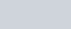Show that the points (−4, −1), (−2, −4) (4, 0) and
Question:

Show that the points (−4, −1), (−2, −4) (4, 0) and (2, 3) are the vertices points of a rectangle.

Solution:

The distance $d$ between two points $\left(x_{1}, y_{1}\right)$ and $\left(x_{2}, y_{2}\right)$ is given by the formula

$d=\sqrt{\left(x_{1}-x_{2}\right)^{2}+\left(y_{1}-y_{2}\right)^{2}}$

In a rectangle, the opposite sides are equal in length. The diagonals of a rectangle are also equal in length.

Here the four points are A(4,1), B(2,4), C(4,0) and D(2,3).

First let us check the length of the opposite sides of the quadrilateral that is formed by these points.

$A B=\sqrt{(-4+2)^{2}+(-1+4)^{2}}$

$=\sqrt{(-2)^{2}+(3)^{2}}$

$=\sqrt{4+9}$

$A B=\sqrt{13}$

$C D=\sqrt{(4-2)^{2}+(0-3)^{2}}$

$=\sqrt{(2)^{2}+(-3)^{2}}$

$=\sqrt{4+9}$

$C D=\sqrt{13}$

We have one pair of opposite sides equal.

Now, let us check the other pair of opposite sides.

$B C=\sqrt{(-2-4)^{2}+(-4-0)^{2}}$

$=\sqrt{(-6)^{2}+(-4)^{2}}$

$=\sqrt{36+16}$

$B C=\sqrt{52}$

$A D=\sqrt{(-4-2)^{2}+(-1-3)^{2}}$

$=\sqrt{(-6)^{2}+(-4)^{2}}$

$=\sqrt{36+16}$

$A D=\sqrt{52}$

The other pair of opposite sides are also equal. So, the quadrilateral formed by these four points is definitely a parallelogram.

For a parallelogram to be a rectangle we need to check if the diagonals are also equal in length.

$A C=\sqrt{(-4-4)^{2}+(-1-0)^{2}}$

$=\sqrt{(-8)^{2}+(-1)^{2}}$

$=\sqrt{64+1}$

$A C=\sqrt{65}$

$B D=\sqrt{(-2-2)^{2}+(-4-3)^{2}}$

$=\sqrt{(-4)^{2}+(-7)^{2}}$

$=\sqrt{16+49}$

$B D=\sqrt{65}$

Now since the diagonals are also equal we can say that the parallelogram is definitely a rectangle.

Hence we have proved that the quadrilateral formed by the four given points is a.